# ACT Math : How to find the length of the side of of an acute / obtuse isosceles triangle

## Example Questions

### Example Question #15 : Acute / Obtuse Isosceles Triangles

A triangle has a perimeter of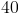inches with one side of lengthinches. If the remaining two sides have lengths in a ratio of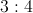, what is length of the shortest side of the triangle?Explanation:

The answer is.

Since we know that the permieter isinches and one side isinches, it can be determined that the remaining two sides must combine to be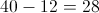inches. The ratio of the remaining two sides iswhich means 3 parts : 4 parts or 7 parts combined. We can then set up the equation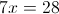, and divide both sides bywhich means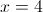. The ratio of the remaining side lengths then becomes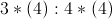or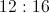. We now know the 3 side lengths are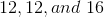.is the shortest side and thus the answer.

### Example Question #231 : Plane Geometry

In the standard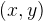coordinate plane, the points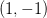and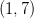form two vertices of an isosceles triangle.  Which of the following points could be the third vertex?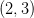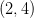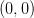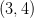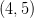Explanation:

To form an isosceles triangle here, we need to create a third vertex whose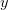coordinate is between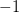and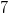.  If a vertex is placed at, the distance fromto this point will be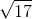. The distance fromto this point will be the same.

### Example Question #232 : Plane Geometry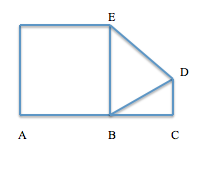Note: Figure is not drawn to scale.

In the figure above, points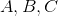are collinear and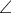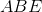is a right angle. If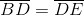and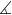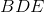is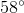, what is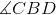?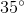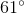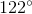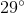Explanation:

Because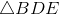is isosceles,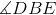equals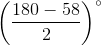or.

We know that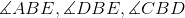add up to, somust equal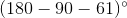or.

### Example Question #233 : Plane Geometry

A light beam of pure white light is aimed horizontally at a prism, which splits the light into two streams that diverge at aangle. The split beams each travel exactly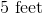from the prism before striking two optic sensors (one for each beam).

What is the distance, in feet, between the two sensors?

Round your final answer to the nearest tenth. Do not round until then.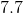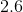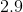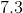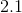Explanation:

This problem can be solved when one realizes that the light beam's split has resulted in an acute isosceles triangle. The triangle as stated has two sides offeet apiece, which meets the requirement for isosceles triangles, and having one angle ofat the vertex where the two congruent sides meet means the other two angles must be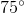and. The missing side connecting the two sensors, therefore, is opposite theangle.

Since we know at least two angles and at least one side of our triangle, we can use the Law of Sines to calculate the remainder. The Law of Sines says that for any triangle with angles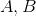and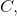and opposite sides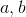and: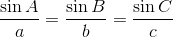.

Plugging in one of ourangles (and its correspondingft side) into this equation, as well as ourangle (and its corresponding unknown side) into this equation gives us: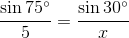Next, cross-multiply:--->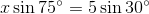Now simplify and solve: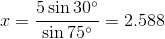Rounding, we see our missing side is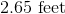long.